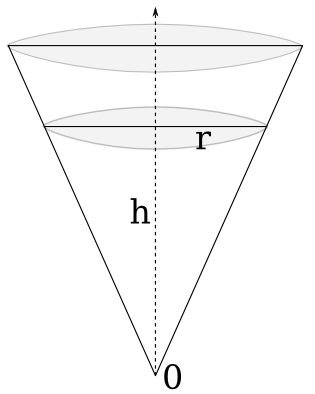## Cocktail dosage in martini glasses: Maths are here to help

New Year’s Eve is getting close, you’d beter be prepared. Let me share a problem I had last day when I decided to make cocktails in martini glasses. How was I suppose to dose my ingredients in such cone-shaped glasses?

Let’s take a first example, The Black Russian recipe is:

2/3 Vodka

1/3  Kahlúa

The problem is that you cannot apply these proportions to the heights of each liquid since the glass is not a cylinder. For a same height, the volume will not be the same, and your cocktail a failure.

Our question is:

What is the ratio between the heights of the two liquids that will make their volumes meet the recipe proportions?

What we have is two volumes vV and vK and their proportion to the total of liquid Vtotal = vV + vK:

And what we want is the ratio hV / Htotal between the height of Vodka and the total height of liquid.Volume of a cone is v = Bh/3, B = π r2 being the base with its radius r.

We then use Thales’ theorem to link any radius r to its corresponding height h:

By using the two last formulas in our volume ratio equation, we get:

What we demonstrated here is a kind of Thales’ theorem (Intercept theorem), but for 3D cones. We obviously have the same kind of result with surfaces of triangles. That’s interesting, that’s a result I never encountered in the past. And it doesn’t only apply to right circular cones, but to any type of cone (pyramids for example), this can be proved by computing the base B using an integral.

The answer to our initial question is then:

That’s right, to have 2/3 of vodka in volume, the height of vodka should be almost 90% of the total height. If the ratio had been 1/2, the height of the first liquid should have been 80% of the total height. It’s not that obvious to me.

Last point, if your cocktail has more than two ingredients (for example a White Russian), I would suggest to always consider cones and not bother with substractions:

1/3 Vodka

1/3  Kahlúa

1/3 Cream

Start by adding 1/3 Kahlúa (70% of total height), then add Vodka until 2/3 of volume (90% of total height) and complete with cream.

Enjoy your cocktails, and happy new year!